### Home > A2C > Chapter Ch4 > Lesson 4.1.3 > Problem4-40

4-40.
1. Find the vertex of each of the following parabolas by averaging the x-intercepts. Then write each equation in graphing form. Homework Help ✎

1. y = (x − 3)(x − 11)

2. y = (x + 2)(x − 6)

3. y = x2 − 14x + 40

4. y = (x −2)2 − 1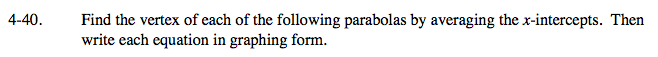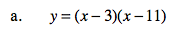x-intercepts: (3, 0) and (11, 0)

Average: x = 7

y = (7 − 3)(7 − 11)

y = −16
(7, −16)
y = (x − 7)2 − 16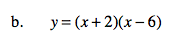(2, −16)
y = (x − 2) − 16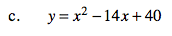Use the same method you used for parts (a) and (b).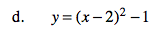(2, −1)

The equation is already in graphing form.